# How to Implement the Omron Host Link Protocol Part 2 – VBA

ACC Omron Host Link VBA

In Part 1, we used VB6 to communicate from the computer to the PLC. We will now use Visual Basic for Applications (VBA) to accomplish the same task. NETComm will be the serial driver and Excel will be the program that we will use. Using Excel we will have access to the Excel Object Model so we can utilize worksheets, ranges etc.

Please refer to Part 1 (How to Implement the Omron PLC Host Link Protocol) for the details of the wiring of the serial port and protocol sequence.

The first step in using VBA is to download and register NETComm.ocx. To use serial communications with VBA you must register the NETComm.ocx driver.

Download the NETComm.ocx file to the c:\ directory.

Call up a dos prompt with administrator authority. (Right click on the cmd.exe program and select run as administrator.)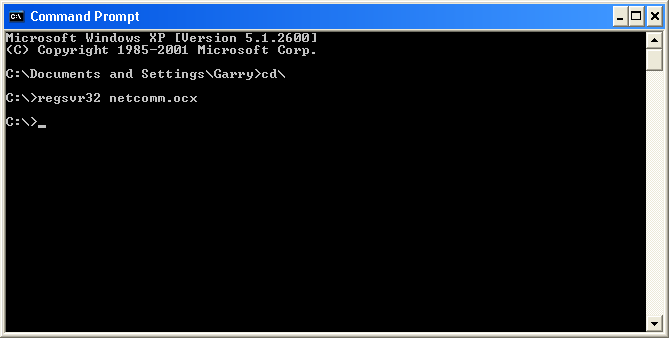Type ‘regsvr32 netcomm.ocx’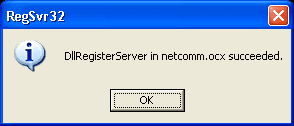An information box will be shown indicating that the NETComm.ocx was registered successfully.

Now open Excel and make the following on Sheet 1.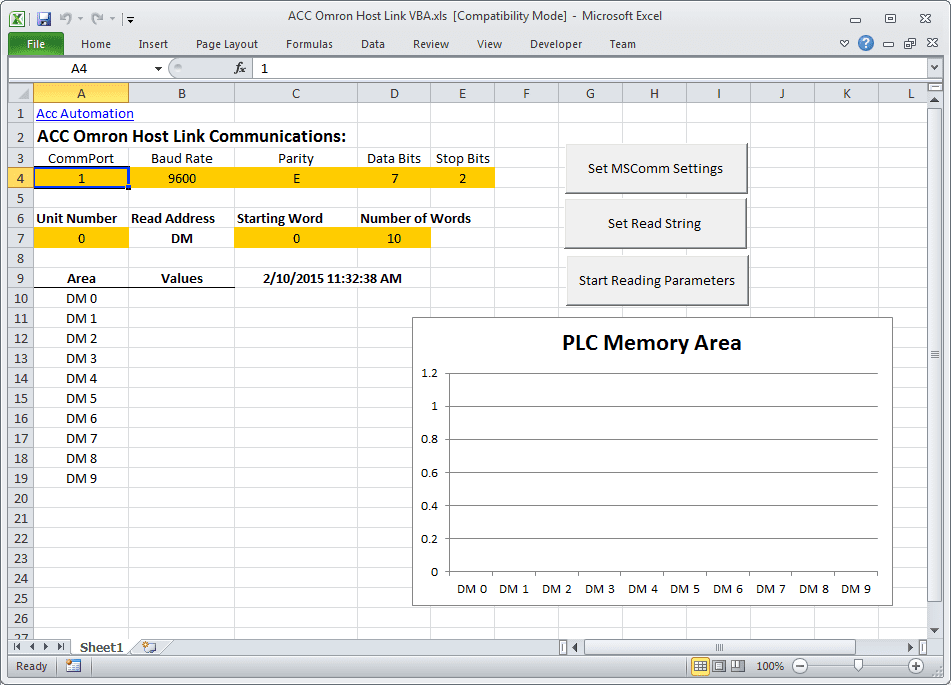Select Developer and then Visual Basic.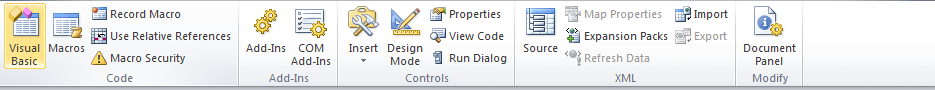If you do not have the Developer option to select, then do the following:
Select File : Options: Customized Ribbon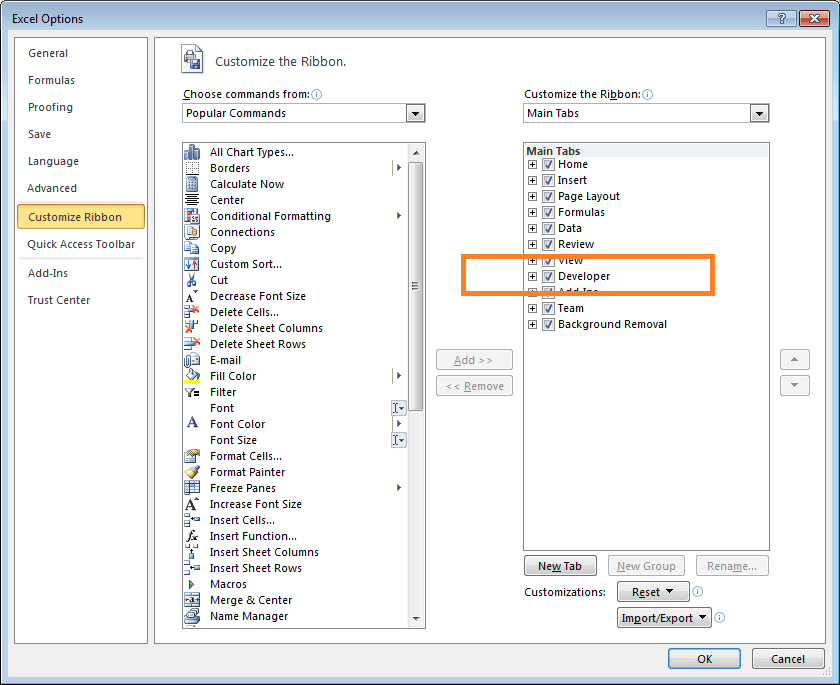Select Developer and hit OKVBA – Visual Basic for Applications will open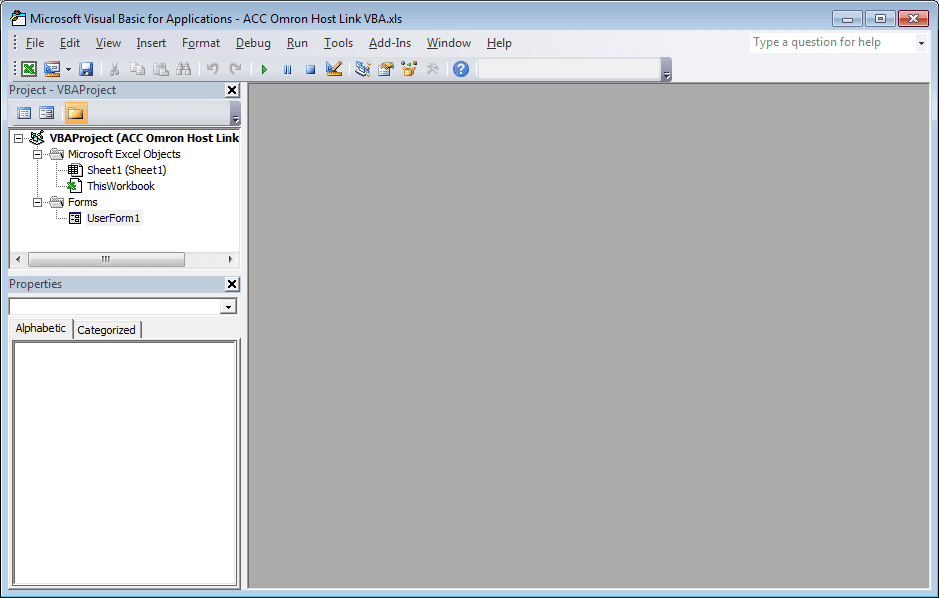Add NETComm1 to UserForm1. If it is not on your Toolbar, then select additional controls and select NETCommOCX.NETComm. Press the OK. Then drag this control onto your form.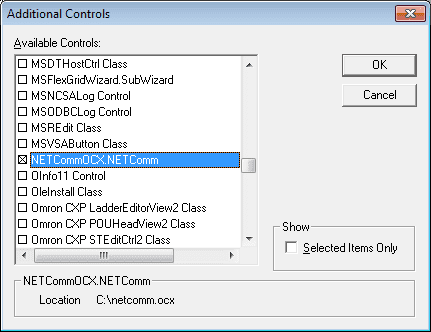Create three command buttons. This can be done by going to Design Mode,  and under the Insert menu you can select Command Buttons.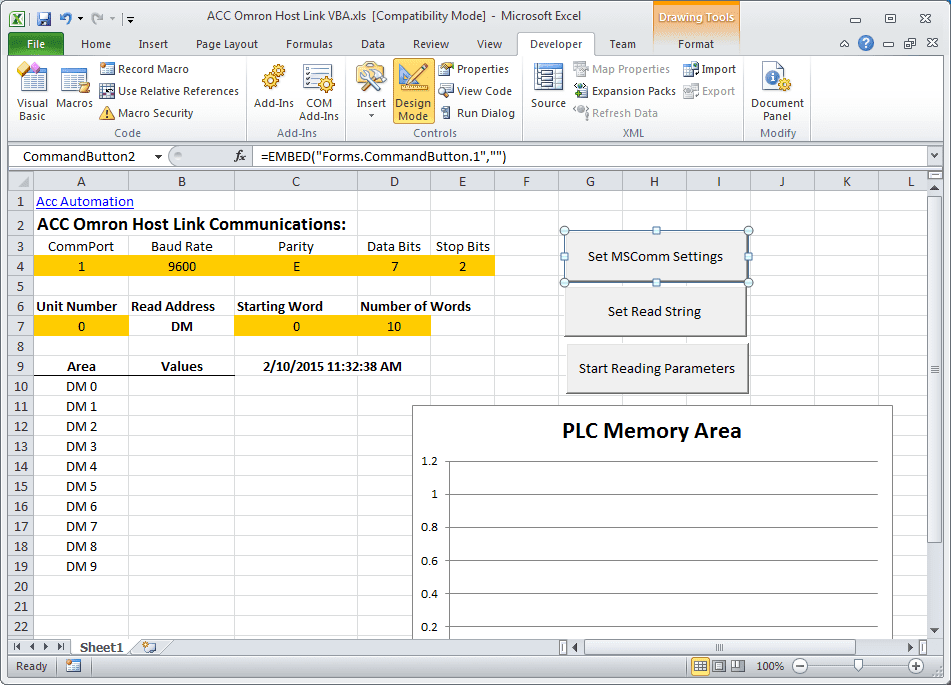Here is the entire code for the application:

```Private T\$ ' Modual Scope for variable
Private TRead\$
Private NumWords
Private startcycle```
```Private Sub CommandButton1_Click()
If NumWords = "" Then
MsgBox "Set Read String"
Exit Sub
End If
If startcycle = 0 Then
startcycle = 1
CommandButton1.BackColor = "&H0000FF00"
Else
startcycle = 0
CommandButton1.BackColor = "&H8000000F"
UserForm1.NETComm1.PortOpen = False ' Close Communication Port
Exit Sub
End If
UserForm1.NETComm1.PortOpen = True ' Open Communication Port
Application.EnableEvents = True
Do
T\$ = TRead\$ ' Reset the Transmitted Information
charreturn = 11 + (NumWords * 4) ' Determine the return characters
GoSub FCS ' Checksum
GoSub communicate ' Get informaiton

'Check returned information and Display
If Mid(rxd\$, 6, 2) = "00" And (Len(rxd\$)) >= charreturn Then
For x = 1 To Sheets("Sheet1").Range("D7")
Sheets("Sheet1").Range("B" & x + 10 - 1) = Mid(rxd\$, x * 4 + 4, 4)
Next 'x
End If```
```    DoEvents ' Do other tasks
'Update the date and time
Sheets("Sheet1").Range("C09") = Format(Date, "YYYY/MM/DD") + "    " + Format(Time, "HH:MM:SS")
Loop While startcycle = 1```
`If startcycle = 0 Then Exit Sub`
```communicate:
rxd\$ = ""
Buffer = T\$ + FCS\$ + "*" + Chr\$(13)
UserForm1.NETComm1.Output = Buffer
Time1 = Now
Timeout = Now + TimeValue("0:00:02")
Do
If Timeout <= Time1 Then GoTo timeoutcom
DoEvents
Time1 = Now()
Loop Until UserForm1.NETComm1.InBufferCount >= charreturn
rxd\$ = UserForm1.NETComm1.InputData
fcs_rxd\$ = Left((Right(rxd\$, 4)), 2)
If Left(rxd\$, 1) = "@" Then
T\$ = Mid(rxd\$, 1, (Len(rxd\$) - 4))
ElseIf Mid(rxd\$, 2, 1) = "@" Then
T\$ = Mid(rxd\$, 2, (Len(rxd\$) - 5))
rxd\$ = Mid(rxd\$, 2, (Len(rxd\$) - 1))
End If
GoSub FCS
If FCS <> fcs_rxd\$ Then
rxd\$ = ""
End If
clearbuffer\$ = UserForm1.NETComm1.InputData
Return```
```FCS:
L = Len(T\$)
A = 0
For J = 1 To L
TJ\$ = Mid\$(T\$, J, 1)
A = Asc(TJ\$) Xor A
Next J
FCS\$ = Hex\$(A)
If Len(FCS\$) = 1 Then FCS\$ = "0" + FCS\$
Return```
```timeoutcom:
If startcycle = 0 Then Exit Sub
clearbuffer\$ = UserForm1.NETComm1.InputData
rxd\$ = ""
Return```
`End Sub`
```Private Sub CommandButton2_Click()
If startcycle = 0 Then
UserForm1.NETComm1.CommPort = Sheets("Sheet1").Range("A4")
UserForm1.NETComm1.Settings = Sheets("Sheet1").Range("B4") & "," & Sheets("Sheet1").Range("C4") & "," & Sheets("Sheet1").Range("D4") & "," & Sheets("Sheet1").Range("E4")
MsgBox UserForm1.NETComm1.Settings
Else
MsgBox "Stop Reading Parameters to Set MSComm"
End If
End Sub```
```Private Sub CommandButton3_Click()
If startcycle = 0 Then
Unit = Sheets("Sheet1").Range("A7")
If Len(Unit) < 2 Then Unit = "0" & Unit
StartADD = Sheets("Sheet1").Range("C7")
Do
If Len(StartADD) < 4 Then
StartADD = "0" & StartADD
End If
Loop Until Len(StartADD) = 4
NumWords = Sheets("Sheet1").Range("D7")
Do
If Len(NumWords) < 4 Then
NumWords = "0" & NumWords
End If
Loop Until Len(NumWords) = 4
T\$ = "@" & Unit & "RD" & StartADD & NumWords
TRead\$ = T\$
MsgBox T\$
For x = 1 To Sheets("Sheet1").Range("D7")
Sheets("Sheet1").Range("A" & x + 10 - 1) = "DM " & Sheets("Sheet1").Range("C7") + x - 1
Sheets("Sheet1").Range("B" & x + 10 - 1) = ""
Next 'x
For x = Sheets("Sheet1").Range("D7") To 200
Sheets("Sheet1").Range("A" & x + 10) = ""
Sheets("Sheet1").Range("B" & x + 10) = ""
Next 'x
Else
MsgBox "Stop Reading Parameters to Set Read String"
End If
End Sub```
`Private Sub Worksheet_SelectionChange(ByVal Target As Range)`
`End Sub`

Note: The Chart on Sheet1 is just a selection of the first ten DM areas and insert a bar graph.

Running the program produces the following information: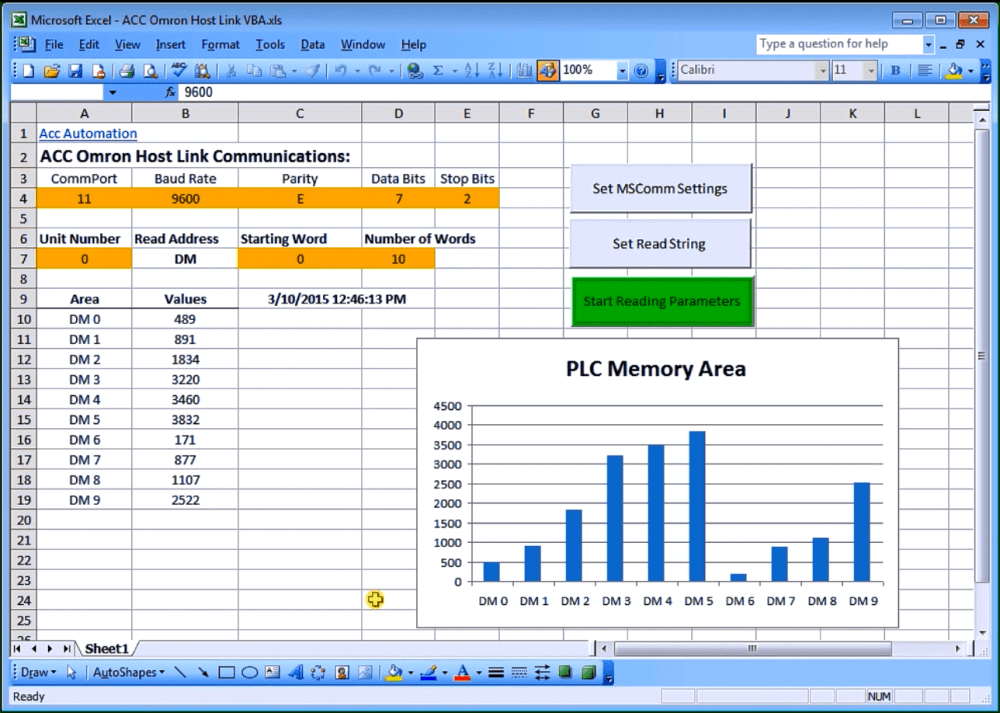Download the excel file ACC Omron Host Link VBA.XLS. This is the complete program mentioned above.

When you open the file it will warn you about macros. This is the VBA application. Press ‘Enable Macros’.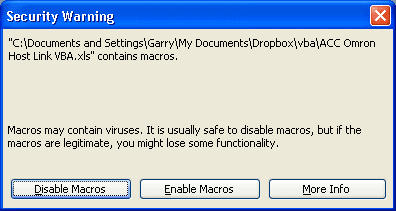When changing the parameters on the screen, you will also get a warning about Active X. This is the NETComm.ocx which was registered above. Press ‘OK’ to run the application.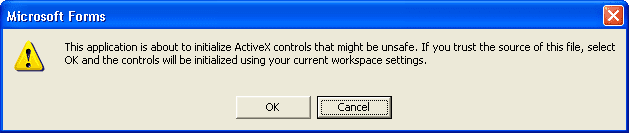Additional Information:
Excel – Conditional Movement of Data

Watch on YouTube : How to Implement the Omron Host Link Protocol Part 2 – VBA
If you have any questions or need further information please contact me.
Thank you,
Garry

If you’re like most of my readers, you’re committed to learning about technology. Numbering systems used in PLC’s are not difficult to learn and understand. We will walk through the numbering systems used in PLCs. This includes Bits, Decimal, Hexadecimal, ASCII and Floating Point.

To get this free article, subscribe to my free email newsletter.

Use the information to inform other people how numbering systems work. Sign up now.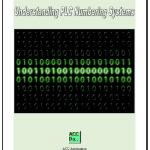The ‘Robust Data Logging for Free’ eBook is also available as a free download. The link is included when you subscribe to ACC Automation.

# How to Implement the Omron PLC Host Link Protocol

Hostlink communication protocol is a method developed by Omron for communication to PLC’s and other equipment. This ASCII based protocol is used over RS232 or RS422/RS485. It is a many to one implementation which means that you can communicate with up to 32 devices back to a master. (1:N) This communication on the industrial floor can control PLC’s, Temperature Controllers, Panel Meters, etc.

Our look at this protocol will include the wiring, setting of RS232 port settings, protocol format and  writing a VB6 program to read information from the PLC. I will also point you links to then store this information into a database and share over an intranet/internet. Lets get started.

Wiring of the communication ports will depend on the equipment purchased. If communicating over 15 meters, it is recommended to switch to RS422 or RS485 connection. However I have seen RS232 runs of 50 meters without an issue. It will depend on your implementation and electrical noise in the plant.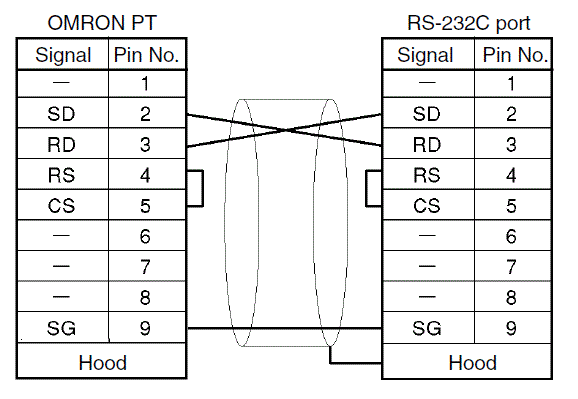The above diagram is the basic communication needed for RS232C. Note that the shield of the communication wire is connected only to one side. This ensures that any noise induced in the communication is filtered to one end.

Settings for RS232C communications are set in a number of ways. Older Omron C**K PLC were set through a series of dip switches. Current Omron SMR1/CPM1 PLC’s are set though data memory locations.
Note: Most of the time, you need to cycle the power or switch to program / run mode for the setting to be activated.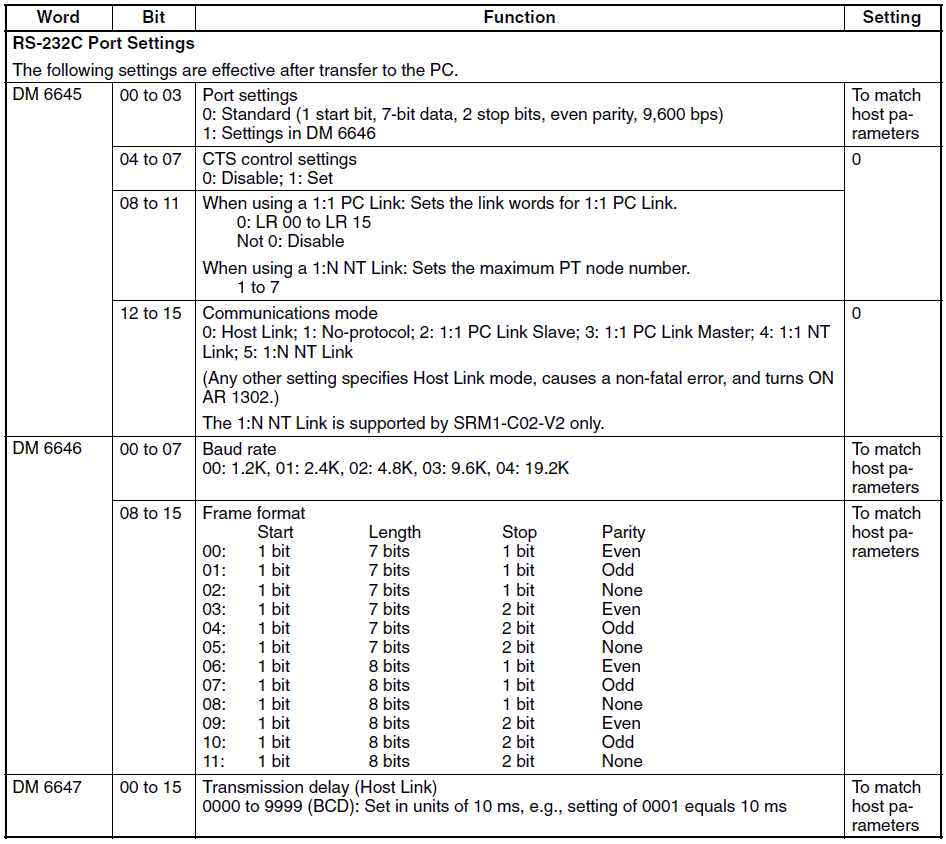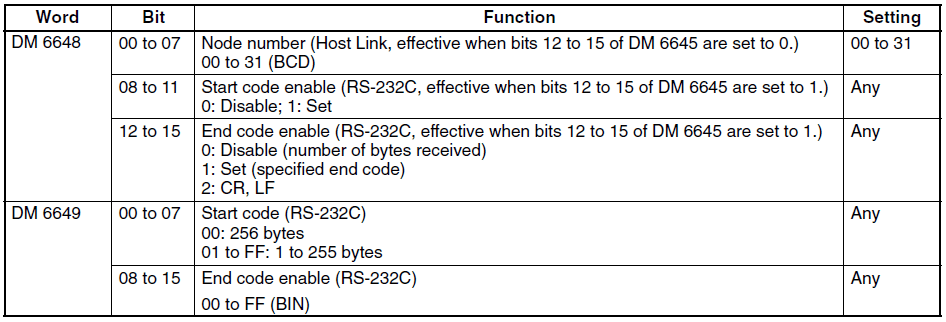I generally tend to leave everything at the default settings: 9600 bps, Even parity, 7 data bits, 1 stop bit. The default host link unit number is 00. (32 max. – 00 – 31)

Protocol Format
Each piece of equipment will have a list of parameters that can be read and written using the HostLink protocol. This can be found in the programming manual of the device. Here are the areas in the CPM1/CPM1A/CPM2A/CPM2C/SRM1(-V2) from the programming manual.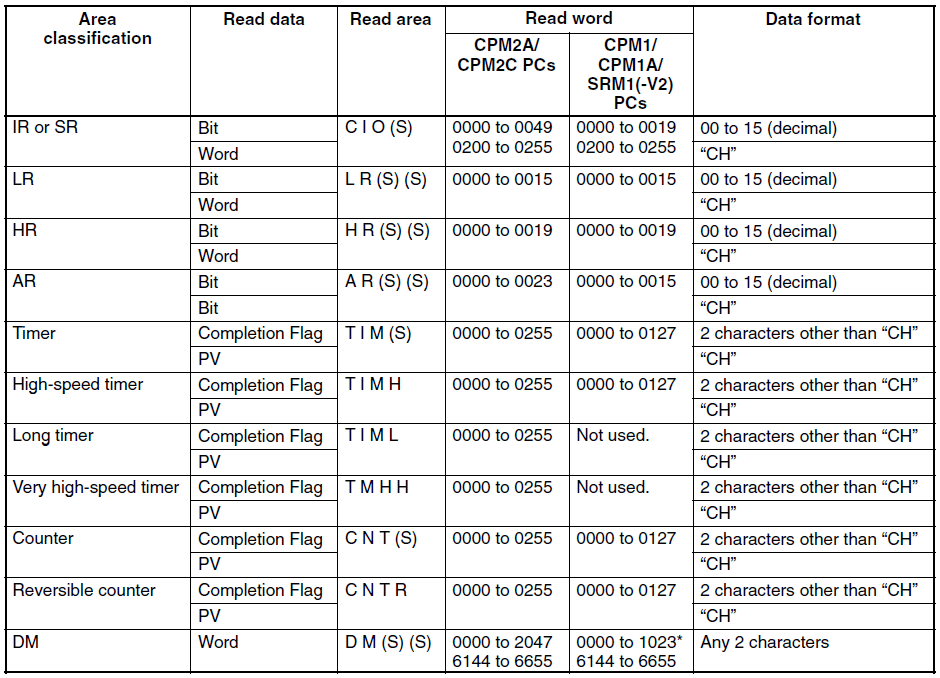Lets take a look at the command to read the DM area. All of the commands and responses will be in an ASCII format.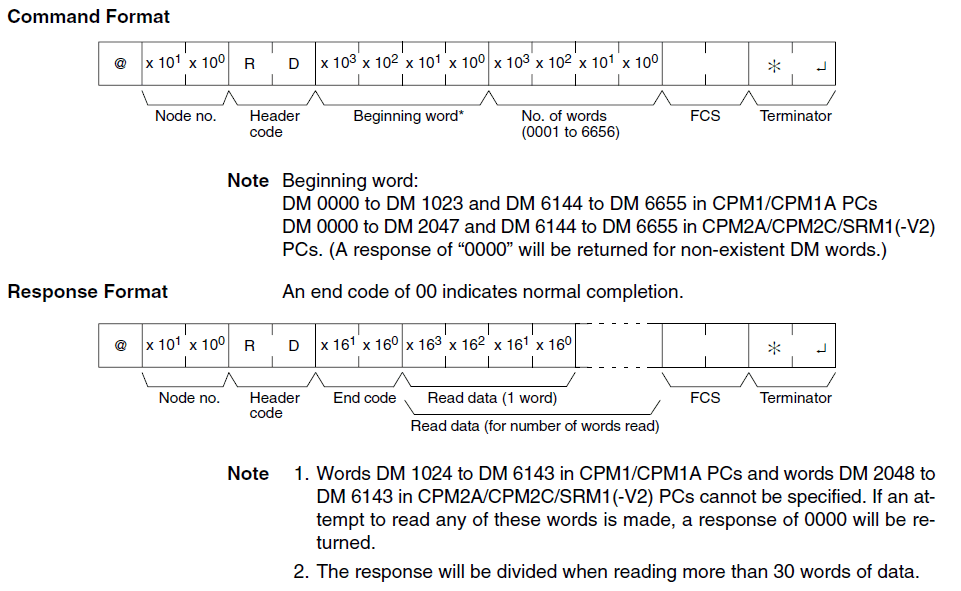The command format begins with a ‘@‘ sign followed by the Node / Unit number that you wish to communicate. Header code is the command in which you with to execute. (RD) This header code will determine the next series of information. In our case the next four digits will be the beginning word followed by the next four digits to indicate the number of words. The next part of the command is the FCS (checksum) calculation. The comparison to this at each end will ensure that the command/response is correct. FCS is a 8 bit data converted into two ASCII characters. The 8 bits are a result of an Exclusive OR performed on the data from the beginning to the end of the text in the frame. In our case this would be performed on the following:

`"@00RD00000010"`

The last part of the command is the terminator. This is an ‘*’ followed by the character for the carriage return. (CHR\$(13))

The response format begins with a ‘@’ sign followed by the Node / Unit number that you are communicating to. The header code is next (RD) followed by the End Code. The end code is a two digit ASCII code that indicates the message response / errors when executing the action. A normal code of ’00’ indicates that everything is fine. See the operation manual for the entire list of end codes for your equipment. The next part of the response depends on the header code executed. In our case it would contain the data requested. The last two parts of the response is the FCS and terminator just like the command format.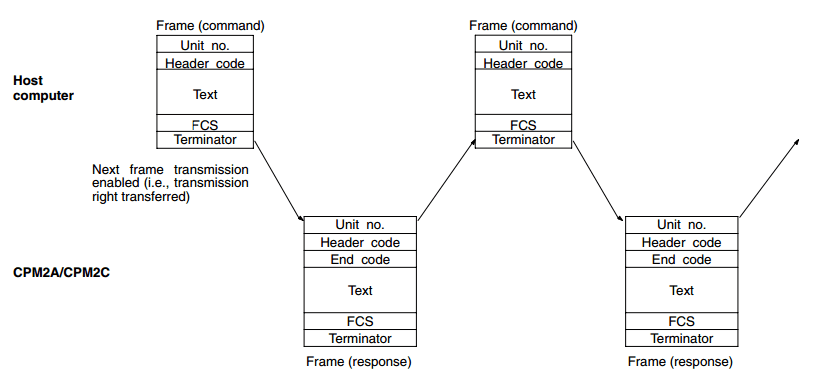The above shows the timing of the command and responses.

Visual Basic VB6 (Example)
Now lets look at an example of reading the first 10 words from the DM area  of an Omron PLC.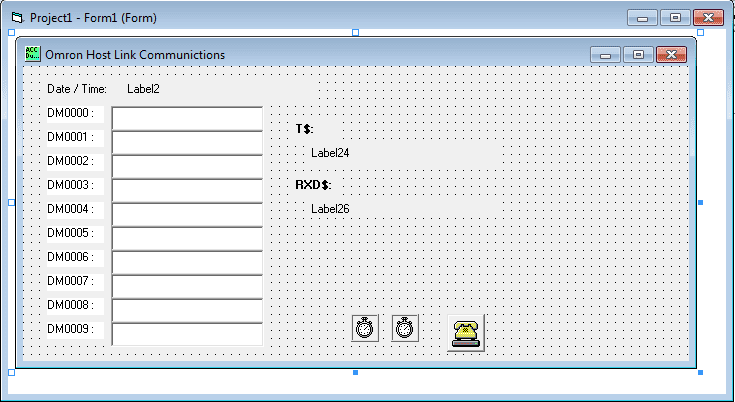The first step is the design the form. You can see that we have our ten DM area words set out to populate with values. We also have a T\$ for transmit. This will show what we are sending to the PLC. The RXD\$ will show what the response will be from the PLC.

The MSComm is used to communicate through the serial ports of the computer. The following is the settings for the communication port.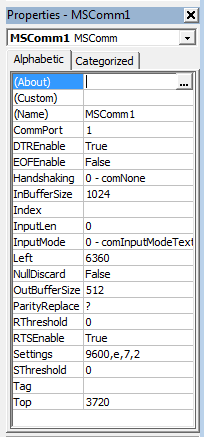Here is the VB6 code for the program:
When the form loads the Date/Time will get updated and Timer1 is enabled. This timer controls the interval in which the commands get executed. (Set to 1 second)

```Private Sub Form_Load()
Label2.Caption = Format(Date, "YYYY/MM/DD") + "    " + Format(Time, "HH:MM:SS")
Timer1.Enabled = True
End Sub```

The following code will open the communication port, set the command format, send the command through the port, receive the response through the port and display the information. It will then close the communication port.

```Private Sub Timer1_Timer()
Timer1.Enabled = False
MSComm1.PortOpen = True
Label2.Caption = Format(Date, "YYYY/MM/DD") + "    " + Format(Time, "HH:MM:SS")```
```'Check DM AREA DM0000 to DM0009 data update
T\$ = "@00RD00000010"
charreturn = 51
GoSub FCS
GoSub communicate```
```'Show Transmit information
Label24.Caption = Buffer
'Show Returned information
Label26.Caption = rxd\$```
```If Mid(rxd\$, 6, 2) = "00" And (Len(rxd\$)) >= charreturn Then
Label4.Caption = Mid(rxd\$, 8, 4)
Label6.Caption = Mid(rxd\$, 12, 4)
Label8.Caption = Mid(rxd\$, 16, 4)
Label10.Caption = Mid(rxd\$, 20, 4)
Label12.Caption = Mid(rxd\$, 24, 4)
Label14.Caption = Mid(rxd\$, 28, 4)
Label16.Caption = Mid(rxd\$, 32, 4)
Label18.Caption = Mid(rxd\$, 36, 4)
Label20.Caption = Mid(rxd\$, 40, 4)
Label22.Caption = Mid(rxd\$, 44, 4)
End If
Timer1.Enabled = True
MSComm1.PortOpen = False
Exit Sub```

The following is the subroutine to communicate. Timer2 is the amount of time to wait before expecting an answer on the communication port. Once the command has been sent a maximum of two seconds is waited for an response. If no response nothing is returned. When the response is obtained, the FCS is checked and if correct the information is returned.

```communicate:
rxd\$ = ""
Buffer = T\$ + FCS\$ + "*" + Chr\$(13)
MSComm1.Output = Buffer
Timer2.Enabled = True
Do
DoEvents
Loop Until Timer2.Enabled = False
If Time > #11:59:50 PM# Then
timeout = #12:00:02 AM#
Else
timeout = DateAdd("s", 2, Time)
End If
MSComm1.InputLen = 0
Do
If timeout <= Time Then GoTo timeoutcom
DoEvents
Loop Until MSComm1.InBufferCount >= charreturn
rxd\$ = MSComm1.Input
fcs_rxd\$ = Left((Right(rxd\$, 4)), 2)
If Left(rxd\$, 1) = "@" Then
T\$ = Mid(rxd\$, 1, (Len(rxd\$) - 4))
ElseIf Mid(rxd\$, 2, 1) = "@" Then
T\$ = Mid(rxd\$, 2, (Len(rxd\$) - 5))
rxd\$ = Mid(rxd\$, 2, (Len(rxd\$) - 1))
End If
GoSub FCS
If FCS <> fcs_rxd\$ Then
rxd\$ = ""
End If
clearbuffer\$ = MSComm1.Input
Return```

This is the FCS (checksum) calculation routine.

```FCS:
L = Len(T\$)
A = 0
For J = 1 To L
TJ\$ = Mid\$(T\$, J, 1)
A = Asc(TJ\$) Xor A
Next J
FCS\$ = Hex\$(A)
If Len(FCS\$) = 1 Then FCS\$ = "0" + FCS\$
Return```

This is the routine that will execute if the response is not received within the time period expected.

```timeoutcom:
clearbuffer\$ = MSComm1.Input
rxd\$ = ""
Return```
`End Sub`

Timer2 was used as a delay before looking for a response after sending the command.

```Private Sub Timer2_Timer()
Timer2.Enabled = False
End Sub```

Here is the code running: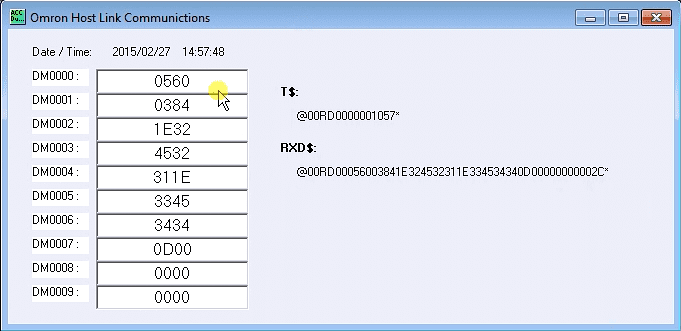Helpful Tips/Links:
– When troubleshooting serial communications it is sometimes helpful to use HyperTerminal. This program will send and receive information in/out of the serial ports.
HostLink Command Generator
HostLink Command Format

Watch on YouTube : How to Implement the Omron PLC Host Link Protocol

If you have any questions, need further information or would like a copy of this program please contact me.
Thank you,
Garry

If you’re like most of my readers, you’re committed to learning about technology. Numbering systems used in PLC’s are not difficult to learn and understand. We will walk through the numbering systems used in PLCs. This includes Bits, Decimal, Hexadecimal, ASCII and Floating Point.

To get this free article, subscribe to my free email newsletter.

Use the information to inform other people how numbering systems work. Sign up now.The ‘Robust Data Logging for Free’ eBook is also available as a free download. The link is included when you subscribe to ACC Automation.